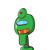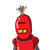# In a block building game, Belén has 56 cubes of 1 unit in side length. Using all of them, she built a rectangular prism with a bas

In a block building game, Belén has 56 cubes of 1 unit in side length. Using all of them, she built a rectangular prism with a base perimeter of 22 unit lengths. What is the height of the prism?

### 2 thoughts on “In a block building game, Belén has 56 cubes of 1 unit in side length. Using all of them, she built a rectangular prism with a bas”

1.Given : In a block building game, Belén has 56 cubes of 1 unit in side length. Using all of them, she built a rectangular prism with a base perimeter of 22 unit lengths. What is the height of the prism ?

we know that,

• Volume of prism = Base Area * height .
• Base Area = L * B
• Base perimeter = 2(L + B)
• Volume of cube = (a)³

Let , height of prism is H unit .

so,

→ Volume of prism = Volume of cubes of 1 unit side length

→ (L * B) * H = 56 * (1)³

→ (L * B) * H = 56

now, given that,

→ 2(L + B) = 22

→ (L + B) = 11

taking, possible values of L and B, and if we assume that , all L , B and H are positive integers , then,

• 1, 10 => H = 5.6 ≠ not a integer .
• 2, 9 => H = 56/18 ≠ not a integer .
• 4, 7 => H => 56/28 = 2 = a integer .

therefore, we can conclude that, values of L and B are 7 and 4 .

hence,

→ 7 * 4 * H = 56

→ H = 2 unit (Ans.)

[ Note :- In question it should be mentioned that H must a integer . otherwise we have to check options. ]

यदि किसी ठोस घन की प्रत्येक भुजा में 150% की वृद्धि

की जाए, तो इसके पृष्ठीय क्षेत्रफल में हुई प्रतिशत वृद्धि

https://brainly.in/question/33888661

किसी कागज की लबाई और चौडाई कमश 33 सेमी. और 16 सेमी. है। इसे इसक

चौडाई के अनुदिश मोड़कर एक बेलन बनाया जाता है। इस बेलन का…

https://brainly.in/question/37931048

2.Given : In a block building game, Belén has 56 cubes of 1 unit in side length. Using all of them, she built a rectangular prism with a base perimeter of 22 unit lengths.

To Find :   height of the prism

8,7,6,4,3,2,1

Solution:

a base perimeter of 22 unit lengths.

Which can be

Base perimeter = 2 ( l + b)

2 (1  + 10)

2 ( 2 + 9)

2 ( 3 + 8)

2 ( 4 + 7)

2 ( 5 + 6)

now l * b * h  = 56

only possible when

4 * 7 * 2 =  56

Hence base area 4 * 7

and height is 2

height of the prism is 2 unit